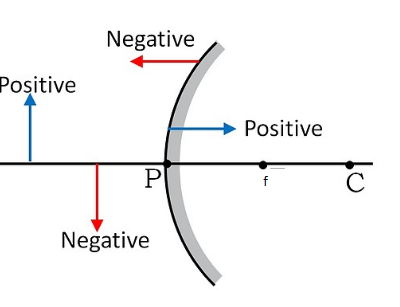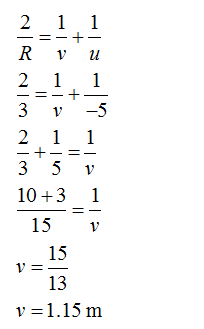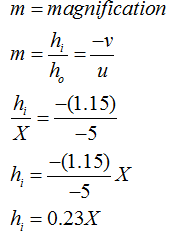# A convex mirror used for rear-view on an automobile has a radius of curvature of 3.00 m. If a bus is located at 5.00 m from this mirror, find the position, nature and size of the image.

Question
1 views

A convex mirror used for rear-view on an automobile has a radius of curvature of 3.00 m. If a bus is located at 5.00 m from this mirror, find the position, nature and size of the image.

check_circle

Step 1Where,

v = image distance from mirror

u = object distance from mirror

f= focal length

The sign convention used in this problem is shown as,The radius of curvature the mirror is 3 m and object distance is 5 m from the mirror.

Let, the height of the object is X m.

We have to find the distance of image, nature of image and height of an image.

Step 2

First, we will find out the position of the image.

The distance of the object is taken as negative.

u = -5 m

R = 3 mThe sign of the image distance is positive it means that the image is formed behind the mirror.

Hence, the nature of the image is virtual.

Step 3

Now, we will find the height of the image.

We assumed that the height of the object is X m.The nature of the image is diminished.

The sign of the height of the image is the same as the height of the object.

So, the nature of the image is erect.

...

### Want to see the full answer?

See Solution

#### Want to see this answer and more?

Solutions are written by subject experts who are available 24/7. Questions are typically answered within 1 hour.*

See Solution
*Response times may vary by subject and question.
Tagged in